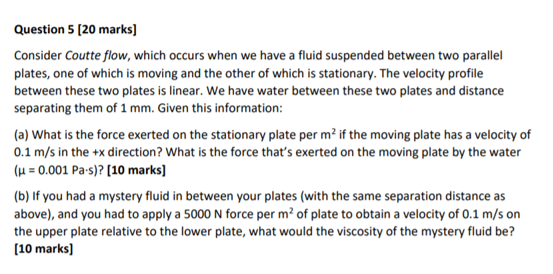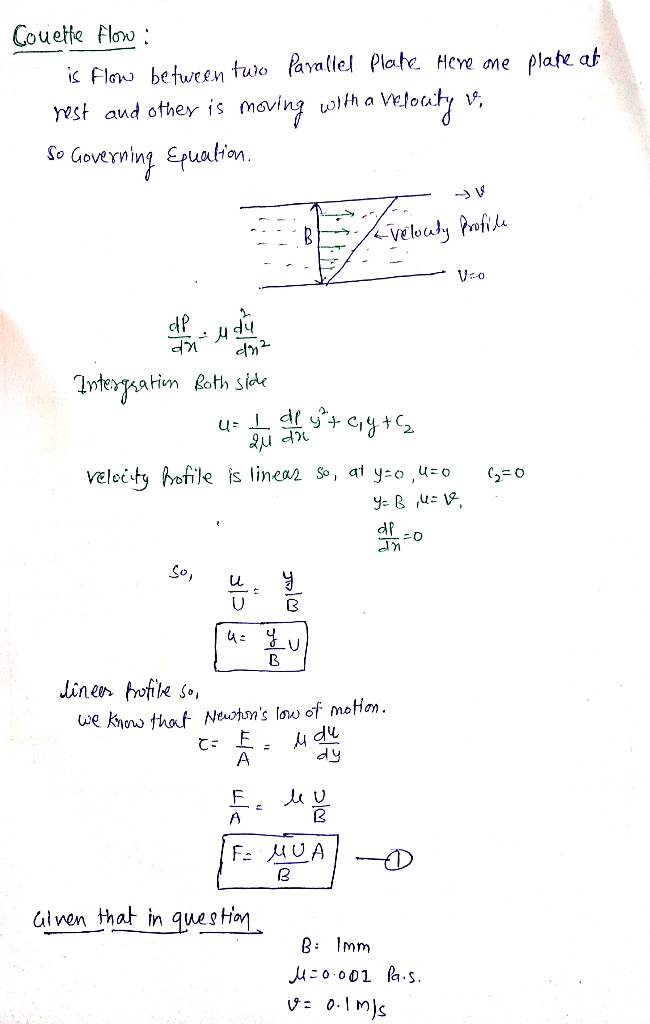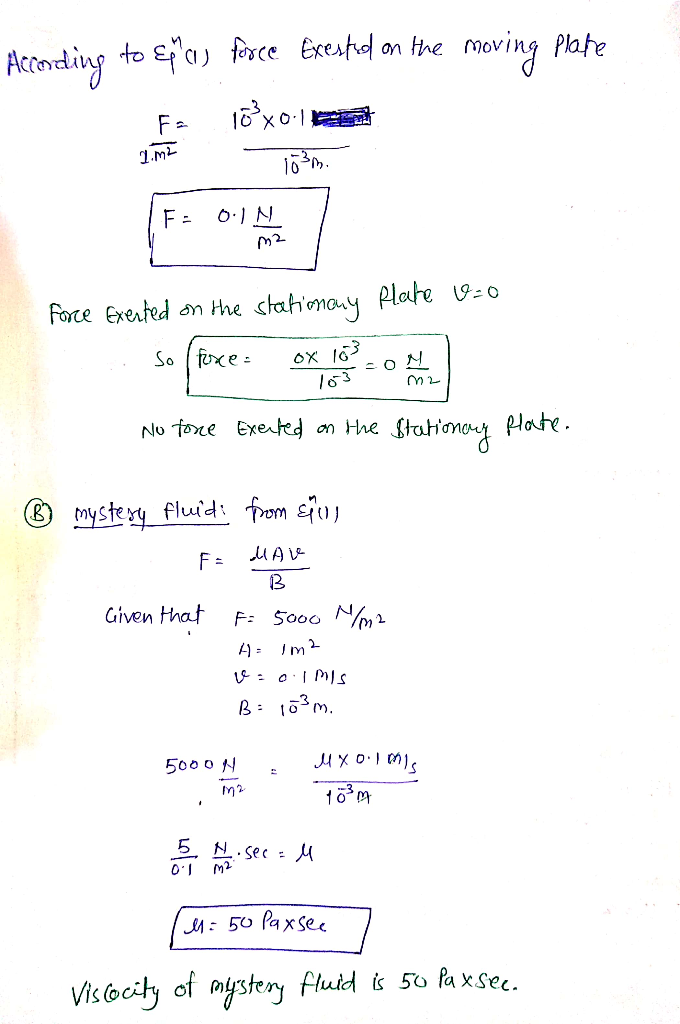# Question 5 [20 marks) Consider Coutte flow, which occurs when we have a fluid suspended between two parallel plates, on...Question 5 [20 marks) Consider Coutte flow, which occurs when we have a fluid suspended between two parallel plates, one of which is moving and the other of which is stationary. The velocity profile between these two plates is linear. We have water between these two plates and distance separating them of 1 mm. Given this information: (a) What is the force exerted on the stationary plate per m2 if the moving plate has a velocity of 0.1 m/s in the +x direction? What is the force that's exerted on the moving plate by the water (με 0.001 Pa-s)? [10 marks] (b) If you had a mystery fluid in between your plates (with the same separation distance as above), and you had to apply a 5000 N force per m2 of plate to obtain a velocity of 0.1 m/s on the upper plate relative to the lower plate, what would the viscosity of the mystery fluid be? [10 marks]##### Add Answer of: Question 5 [20 marks) Consider Coutte flow, which occurs when we have a fluid suspended between two parallel plates, on...
More Homework Help Questions Additional questions in this topic.